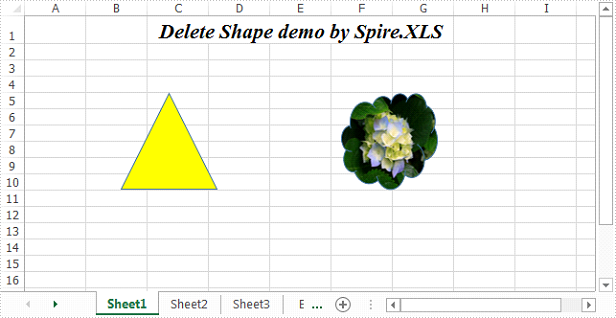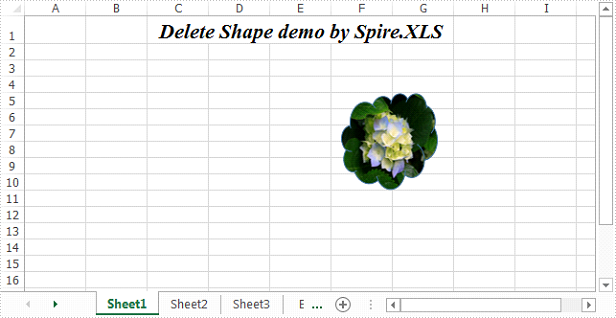# Delete shapes in an Excel Worksheet in C#

Spire.XLS supports to delete a specific shape as well as all shapes in an Excel worksheet. This article demonstrates how to use Spire.XLS to implement this function.

The example file we used for demonstration:Detail steps:

Step 1: Initialize an object of Workbook class and load the Excel file.

```Workbook workbook = new Workbook();
```

Step 2: Get the first worksheet.

```Worksheet sheet = workbook.Worksheets;
```

Step 3: Delete the first shape in the worksheet.

```sheet.PrstGeomShapes.Remove();
```

To delete all shapes from the worksheet:

```for (int i = sheet.PrstGeomShapes.Count-1; i >= 0; i--)
{
sheet.PrstGeomShapes[i].Remove();
}
```

Step 4: Save the file.

```workbook.SaveToFile("DeleteShape.xlsx", ExcelVersion.Version2013);
```

Screenshot:Full code:

```using Spire.Xls;
namespace DeleteShape
{
class Program
{
static void Main(string[] args)
{
//Initialize an object of Workbook class
Workbook workbook = new Workbook();

//Get the first worksheet
Worksheet sheet = workbook.Worksheets;

//Delete the first shape in the worksheet
sheet.PrstGeomShapes.Remove();

//Delete all shapes in the worksheet
//for (int i = sheet.PrstGeomShapes.Count-1; i >= 0; i--)
//{
//    sheet.PrstGeomShapes[i].Remove();
//}

workbook.SaveToFile("DeleteShape.xlsx", ExcelVersion.Version2013);
}
}
}
```